Question

# calculate the amount of heat in KJ that is required to heat 25.0g of ice from...

calculate the amount of heat in KJ that is required to heat 25.0g of ice from -25c to 105degrees celciusto 105 degrees celcius in a close vessel and sketch a heating curve for the process.The specific heat of ice is 2.11J/(g.degrees celcius);4.18J/(g.degrees celcius for water2.00J/g.degrees celcius.DH for water is 6.01KJ/mol;DH for water 40.67KJ/mol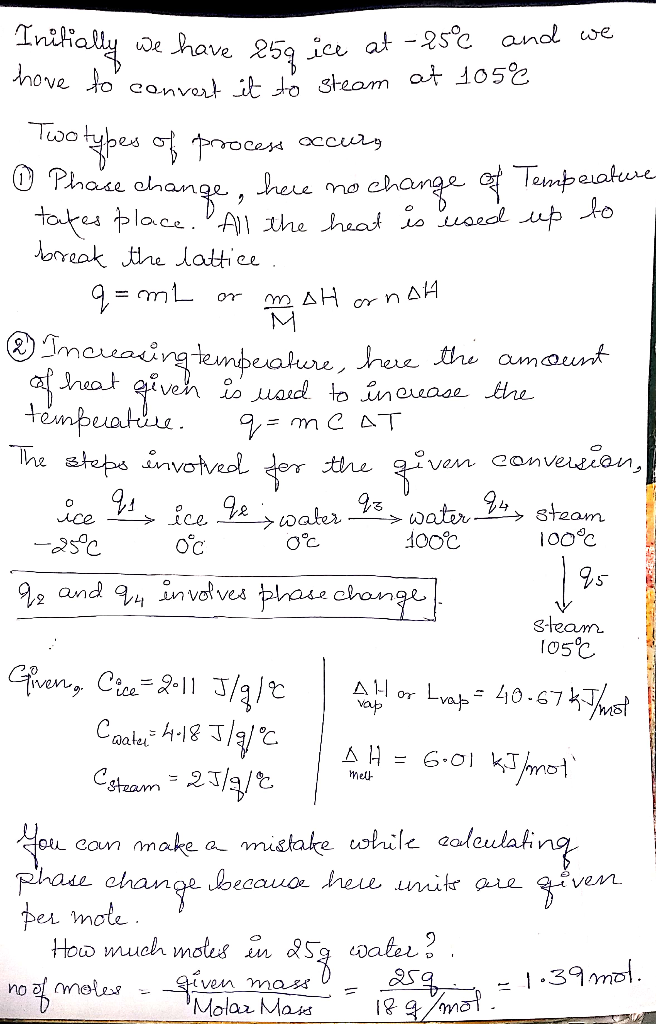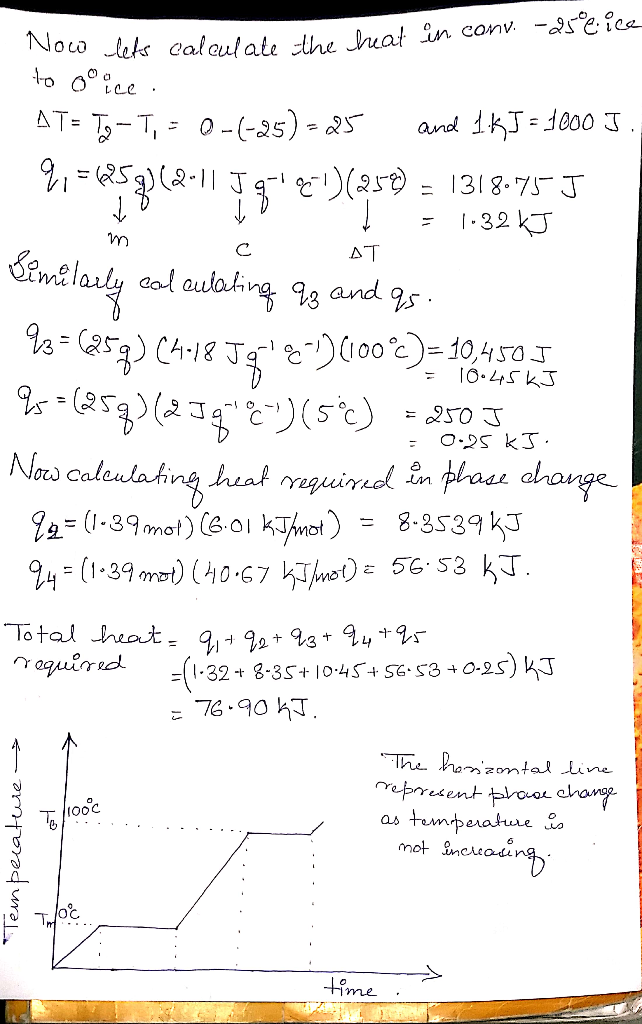#### Earn Coins

Coins can be redeemed for fabulous gifts.

Similar Homework Help Questions
• ### Calculate the amount of heat in kJ that is required to heat 20.0 g of ice...

Calculate the amount of heat in kJ that is required to heat 20.0 g of ice from -25 ºC to 90 ºC, and sketch a heating curve for the process. The specific heat of ice is 2.11 J/( g. ºC); water 4.18 J/( g. ºC) and the ΔHfus for water is 6.01 kJ/mol

• ### 11. The following would be required for calculations of heat flow in which of the heating...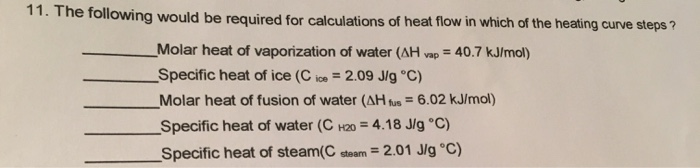11. The following would be required for calculations of heat flow in which of the heating curve steps ? Molar heat of vaporization of water (AH vap = 40.7 kJ/mol) Specific heat of ice (Cice = 2.09 J/g °C) Molar heat of fusion of water (AH fus = 6.02 kJ/mol) Specific heat of water (C H20 = 4.18 J/g °C) Specific heat of steam(C steam = 2.01 J/g °C) Heating Curve for Water Degrees Celsius -50+ 0 400 800 1200...

• ### How much heat is required to convert 155g of ice at -10°C into water vapor at...

How much heat is required to convert 155g of ice at -10°C into water vapor at 110°C? sice = 2.09J/g·°C, swater = 4.18J/g·°C, svapor = 1.84J/g·°C, ΔHfusion = 6.01kJ/mol, ΔHvap = 40.67kJ/mol

• ### Answer the Q.'s I the spaces below. 1. 'Antifreeze' (ethylene glycol, (HOCH,CH,OH)) is much more viscous...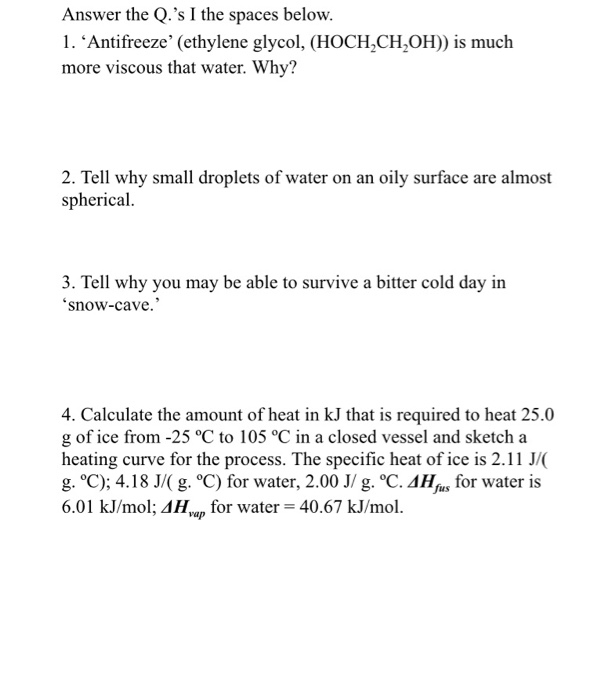Answer the Q.'s I the spaces below. 1. 'Antifreeze' (ethylene glycol, (HOCH,CH,OH)) is much more viscous that water. Why? 2. Tell why small droplets of water on an oily surface are almost spherical 3. Tell why you may be able to survive a bitter cold day in 'snow-cave. 4. Calculate the amount of heat in kJ that is required to heat 25.0 g of ice from -25 °C to 105 °C in a closed vessel and sketch a heating curve...

• ### How much heat required to convert 36 grams (2 moles) of ice at 0 degrees C...

How much heat required to convert 36 grams (2 moles) of ice at 0 degrees C to liquid water at 50 degrees C? delta-H of fusion for H2O is 6.02 kJ/mol and the heat capacity of liquid water is 4.18J/g degrees C. A) 12 kJ B) 7524 J C) 8728 J D) 19564 J

• ### The following table provides the specific heat and enthalpy changes for water and ice Substance Specific...

The following table provides the specific heat and enthalpy changes for water and ice Substance Specific heat [J/(g⋅∘C)][J/(g⋅∘C)] ΔHΔH (kJ/mol)(kJ/mol) water 4.18 44.0 ice 2.01 6.01 a) Calculate the enthalpy change, ΔHΔHDeltaH, for the process in which 42.3 gg of water is converted from liquid at 3.2 ∘C∘C to vapor at 25.0 ∘C∘C . For water, ΔHvapΔHvapH = 44.0 kJ/molkJ/mol at 25.0 ∘C∘C and CsCsC_s = 4.18  J/(g⋅∘C) J/(g⋅∘C) for H2O(l)H2O(l). Express your answer to three significant figures and include the...

• ### Calculate the heat required in Joules to convert 18.0 grams of water ice at a temperature...

Calculate the heat required in Joules to convert 18.0 grams of water ice at a temperature of -20° C to liquid water at the normal boiling point of water. Given: -specific heat of ice = 2.09 J/g°C -specific heat of liquid water = 4.184 J/g°C -specific heat of water vapor = 2.03 J/g°C -molar heat of fusion of water = 6.02 kJ/mol -molar heat of vaporization of water = 40.7 kJ/mol

• ### Water will rise up into a capillary tube. Why, and how is this phenomenon important in...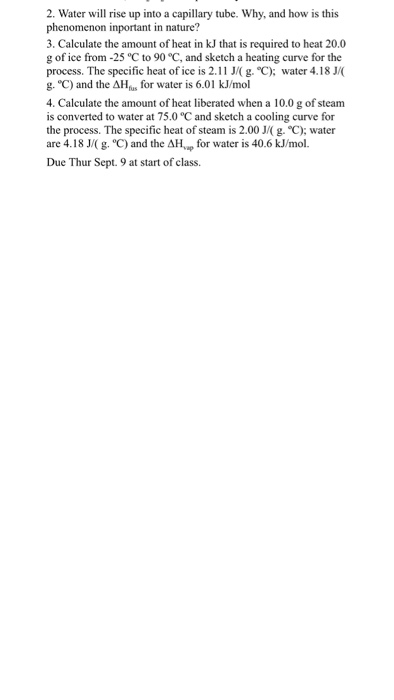Water will rise up into a capillary tube. Why, and how is this phenomenon important in nature? Calculate the amount of heat in kJ that is required to heat 20.0 g of ice from -25 degree C to 90 degree C, and sketch a heating curve for the process. The specific heat of ice is 2.11 J/(g. degree C); water 4.18 J/(g. degree C) and the Delta H_fus for water is 6.01 kJ/mol Calculate the amount of heat liberated when...

• ### Methane, CH_4, is a gas room temperature but dichloromethane, CH_2Cl_2 is a liquid. Why? Water will...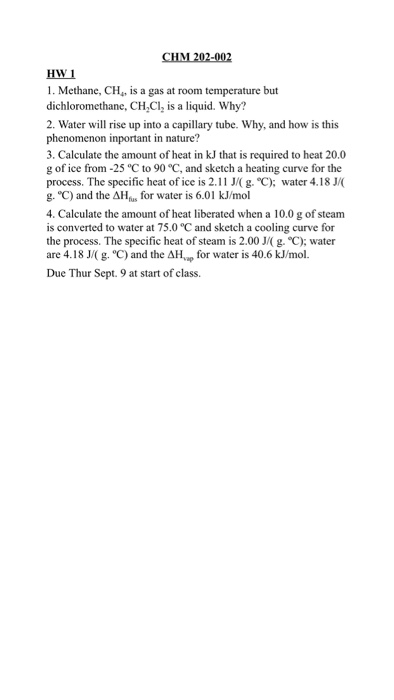Methane, CH_4, is a gas room temperature but dichloromethane, CH_2Cl_2 is a liquid. Why? Water will rise up into a capillary tube. Why, and how is this phenomenon important in nature? Calculate the amount of heat in kJ that is required to heat 20.0g of ice from -25 degree C to 90 degree C, and sketch a heating curve for the process. The specific heat of ice is 2.11 J/(g, degree C); water 4.18 J/(g, degree C) and the Delta...

• ### 8. Calculate the amount of heat (in kJ) required to heat 1.01 kilogram of water from...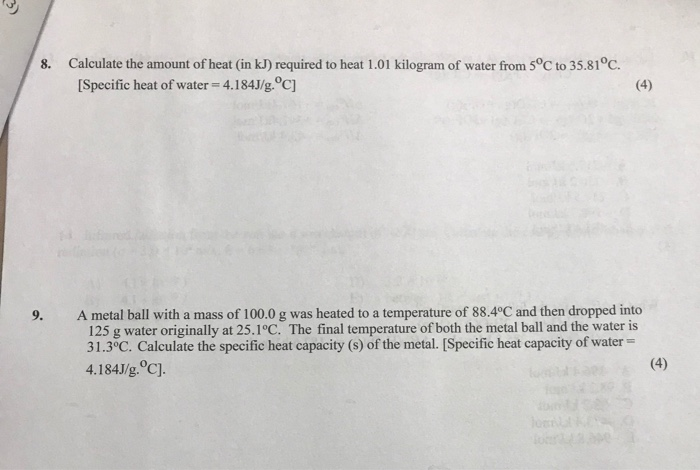8. Calculate the amount of heat (in kJ) required to heat 1.01 kilogram of water from 5°C to 35.81°C. [Specific heat of water-4.184J/g.°C] A metal ball with a mass of 100.0 g was heated to a temperature of 88.4°C and then dropped into 125 g water originally at 25.1°C. The final temperature of both the metal ball and the water is 31.3°C. Calculate the specific heat capacity (s) of the metal. [Specific heat capacity of water = 4.184J/g.°c).